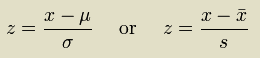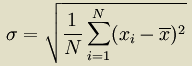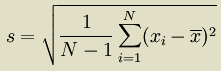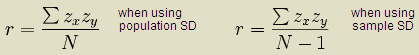# zscores manpage

### COMMAND

zscores -- Calculate standard scores of numerical data spines.

### SYNOPSIS

zscores [input[> output]

### OPTIONS

 -f # Select spine # in the input for processing. The first non-**kern spine is the default. -s Display measured mean and standard deviations of input data only (not the resulting z-scores). -i Display only the input data as a single-column list of numbers. --raw Display the output data as a single column of numbers. -S Suppress the printing of the mean and standard deviation in output. -a Append analysis data to input contents. -p Prepend analysis data to input contents. --replace Substitute analysis data in original data position in the input data. --full Display full location of extracted/processed numbers in input. -m # Use the specified mean when calculating the z-scores. -d # Use the specified standard deviation when calculating the z-scores. --sample Use the sample standard deviation rather than the population standard deviation.

### DESCRIPTION

zscores converts spines of numerical data into values equivalent to the standard deviation scale by shifting the average value of the sequence to zero, and dividing by the standard deviation to generate standard scores. This allows separate sets of numbers with different ranges to be compared more directly.

To convert a set of numbers to their equivalent z-scores, the following equation is used:By default zscores uses the population standard deviation:If you need to use the sample standard deviation:you can use the --sample option. The sample standard deviation is used when you have only a small number of values which probably do not match the actual mean and standard deviation if you were to have a larger set of numbers generated in the same manner (the population). Using a small subset of a population usually results in a less accurate estimate of the underlying mean and standard deviation of the entire population which the sample standard deviation tries to correct by increasing the standard deviation slightly.

Z-scores can be used to calculate Pearson correlation:where r is called the "r-value" (i.e., the Pearson correlation) between two sequences; zx are the z-scores for the first sequence to compare, zy for the other sequence, and N is the length of the two sequences.

### ONLINE DATA

Input arguments or piped data which are expected to be Humdrum files can also be web addresses. For example, if a program can process files like this:
`       program file.krn`
It can also read the data over the web:
`       program http://www.some-computer.com/some-directory/file.krn`
Piped data works in a somewhat similar manner:
`       cat file.krn | program`
is equivalent to a web file using ths form:
`       echo http://www.some-computer.com/some-directory/file.krn | program`

Besides the http:// protocol, there is another special resource indicator prefix called humdrum:// which downloads data from the kernscores website. For example, using the URI humdrum://brandenburg/bwv1046a.krn:

`      program humdrum://brandenburg/bwv1046a.krn`
Which is found in the Musedata Bach Brandenburg Concerto collection.

This online-access of Humdrum data can also interface with the classical Humdrum Toolkit commands by using humcat to download the data from the kernscores website. For example, try the command pipeline:

`      humcat humdrum://brandenburg/bwv1046a.krn | census -k`

```